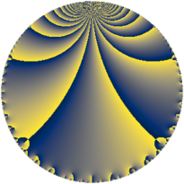# Properties

 Label 300.3.b.aLevel $300$ Weight $3$ Character orbit 300.b Analytic conductor $8.174$ Analytic rank $0$ Dimension $2$ CM discriminant -3 Inner twists $4$

# Related objects

## Newspace parameters

 Level: $$N$$ $$=$$ $$300 = 2^{2} \cdot 3 \cdot 5^{2}$$ Weight: $$k$$ $$=$$ $$3$$ Character orbit: $$[\chi]$$ $$=$$ 300.b (of order $$2$$, degree $$1$$, not minimal)

## Newform invariants

 Self dual: no Analytic conductor: $$8.17440793081$$ Analytic rank: $$0$$ Dimension: $$2$$ Coefficient field: $$\Q(\sqrt{-1})$$ Defining polynomial: $$x^{2} + 1$$ Coefficient ring: $$\Z[a_1, \ldots, a_{7}]$$ Coefficient ring index: $$1$$ Twist minimal: no (minimal twist has level 12) Sato-Tate group: $\mathrm{U}(1)[D_{2}]$

## $q$-expansion

Coefficients of the $$q$$-expansion are expressed in terms of $$i = \sqrt{-1}$$. We also show the integral $$q$$-expansion of the trace form.

 $$f(q)$$ $$=$$ $$q + 3 i q^{3} + 2 i q^{7} -9 q^{9} +O(q^{10})$$ $$q + 3 i q^{3} + 2 i q^{7} -9 q^{9} + 22 i q^{13} -26 q^{19} -6 q^{21} -27 i q^{27} -46 q^{31} + 26 i q^{37} -66 q^{39} + 22 i q^{43} + 45 q^{49} -78 i q^{57} + 74 q^{61} -18 i q^{63} + 122 i q^{67} + 46 i q^{73} + 142 q^{79} + 81 q^{81} -44 q^{91} -138 i q^{93} + 2 i q^{97} +O(q^{100})$$ $$\operatorname{Tr}(f)(q)$$ $$=$$ $$2q - 18q^{9} + O(q^{10})$$ $$2q - 18q^{9} - 52q^{19} - 12q^{21} - 92q^{31} - 132q^{39} + 90q^{49} + 148q^{61} + 284q^{79} + 162q^{81} - 88q^{91} + O(q^{100})$$

## Character values

We give the values of $$\chi$$ on generators for $$\left(\mathbb{Z}/300\mathbb{Z}\right)^\times$$.

 $$n$$ $$101$$ $$151$$ $$277$$ $$\chi(n)$$ $$-1$$ $$1$$ $$-1$$

## Embeddings

For each embedding $$\iota_m$$ of the coefficient field, the values $$\iota_m(a_n)$$ are shown below.

For more information on an embedded modular form you can click on its label.

Label $$\iota_m(\nu)$$ $$a_{2}$$ $$a_{3}$$ $$a_{4}$$ $$a_{5}$$ $$a_{6}$$ $$a_{7}$$ $$a_{8}$$ $$a_{9}$$ $$a_{10}$$
149.1
 − 1.00000i 1.00000i
0 3.00000i 0 0 0 2.00000i 0 −9.00000 0
149.2 0 3.00000i 0 0 0 2.00000i 0 −9.00000 0
 $$n$$: e.g. 2-40 or 990-1000 Significant digits: Format: Complex embeddings Normalized embeddings Satake parameters Satake angles

## Inner twists

Char Parity Ord Mult Type
1.a even 1 1 trivial
3.b odd 2 1 CM by $$\Q(\sqrt{-3})$$
5.b even 2 1 inner
15.d odd 2 1 inner

## Twists

By twisting character orbit
Char Parity Ord Mult Type Twist Min Dim
1.a even 1 1 trivial 300.3.b.a 2
3.b odd 2 1 CM 300.3.b.a 2
4.b odd 2 1 1200.3.c.c 2
5.b even 2 1 inner 300.3.b.a 2
5.c odd 4 1 12.3.c.a 1
5.c odd 4 1 300.3.g.b 1
12.b even 2 1 1200.3.c.c 2
15.d odd 2 1 inner 300.3.b.a 2
15.e even 4 1 12.3.c.a 1
15.e even 4 1 300.3.g.b 1
20.d odd 2 1 1200.3.c.c 2
20.e even 4 1 48.3.e.a 1
20.e even 4 1 1200.3.l.b 1
35.f even 4 1 588.3.c.c 1
35.k even 12 2 588.3.p.b 2
35.l odd 12 2 588.3.p.c 2
40.i odd 4 1 192.3.e.b 1
40.k even 4 1 192.3.e.a 1
45.k odd 12 2 324.3.g.b 2
45.l even 12 2 324.3.g.b 2
55.e even 4 1 1452.3.e.b 1
60.h even 2 1 1200.3.c.c 2
60.l odd 4 1 48.3.e.a 1
60.l odd 4 1 1200.3.l.b 1
80.i odd 4 1 768.3.h.a 2
80.j even 4 1 768.3.h.b 2
80.s even 4 1 768.3.h.b 2
80.t odd 4 1 768.3.h.a 2
105.k odd 4 1 588.3.c.c 1
105.w odd 12 2 588.3.p.b 2
105.x even 12 2 588.3.p.c 2
120.q odd 4 1 192.3.e.a 1
120.w even 4 1 192.3.e.b 1
165.l odd 4 1 1452.3.e.b 1
180.v odd 12 2 1296.3.q.b 2
180.x even 12 2 1296.3.q.b 2
240.z odd 4 1 768.3.h.b 2
240.bb even 4 1 768.3.h.a 2
240.bd odd 4 1 768.3.h.b 2
240.bf even 4 1 768.3.h.a 2

By twisted newform orbit
Twist Min Dim Char Parity Ord Mult Type
12.3.c.a 1 5.c odd 4 1
12.3.c.a 1 15.e even 4 1
48.3.e.a 1 20.e even 4 1
48.3.e.a 1 60.l odd 4 1
192.3.e.a 1 40.k even 4 1
192.3.e.a 1 120.q odd 4 1
192.3.e.b 1 40.i odd 4 1
192.3.e.b 1 120.w even 4 1
300.3.b.a 2 1.a even 1 1 trivial
300.3.b.a 2 3.b odd 2 1 CM
300.3.b.a 2 5.b even 2 1 inner
300.3.b.a 2 15.d odd 2 1 inner
300.3.g.b 1 5.c odd 4 1
300.3.g.b 1 15.e even 4 1
324.3.g.b 2 45.k odd 12 2
324.3.g.b 2 45.l even 12 2
588.3.c.c 1 35.f even 4 1
588.3.c.c 1 105.k odd 4 1
588.3.p.b 2 35.k even 12 2
588.3.p.b 2 105.w odd 12 2
588.3.p.c 2 35.l odd 12 2
588.3.p.c 2 105.x even 12 2
768.3.h.a 2 80.i odd 4 1
768.3.h.a 2 80.t odd 4 1
768.3.h.a 2 240.bb even 4 1
768.3.h.a 2 240.bf even 4 1
768.3.h.b 2 80.j even 4 1
768.3.h.b 2 80.s even 4 1
768.3.h.b 2 240.z odd 4 1
768.3.h.b 2 240.bd odd 4 1
1200.3.c.c 2 4.b odd 2 1
1200.3.c.c 2 12.b even 2 1
1200.3.c.c 2 20.d odd 2 1
1200.3.c.c 2 60.h even 2 1
1200.3.l.b 1 20.e even 4 1
1200.3.l.b 1 60.l odd 4 1
1296.3.q.b 2 180.v odd 12 2
1296.3.q.b 2 180.x even 12 2
1452.3.e.b 1 55.e even 4 1
1452.3.e.b 1 165.l odd 4 1

## Hecke kernels

This newform subspace can be constructed as the intersection of the kernels of the following linear operators acting on $$S_{3}^{\mathrm{new}}(300, [\chi])$$:

 $$T_{7}^{2} + 4$$ $$T_{11}$$

## Hecke characteristic polynomials

$p$ $F_p(T)$
$2$ $$T^{2}$$
$3$ $$9 + T^{2}$$
$5$ $$T^{2}$$
$7$ $$4 + T^{2}$$
$11$ $$T^{2}$$
$13$ $$484 + T^{2}$$
$17$ $$T^{2}$$
$19$ $$( 26 + T )^{2}$$
$23$ $$T^{2}$$
$29$ $$T^{2}$$
$31$ $$( 46 + T )^{2}$$
$37$ $$676 + T^{2}$$
$41$ $$T^{2}$$
$43$ $$484 + T^{2}$$
$47$ $$T^{2}$$
$53$ $$T^{2}$$
$59$ $$T^{2}$$
$61$ $$( -74 + T )^{2}$$
$67$ $$14884 + T^{2}$$
$71$ $$T^{2}$$
$73$ $$2116 + T^{2}$$
$79$ $$( -142 + T )^{2}$$
$83$ $$T^{2}$$
$89$ $$T^{2}$$
$97$ $$4 + T^{2}$$